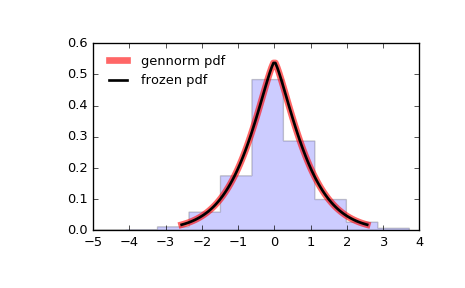# scipy.stats.gennorm¶

scipy.stats.gennorm = <scipy.stats._continuous_distns.gennorm_gen object>[source]

A generalized normal continuous random variable.

As an instance of the rv_continuous class, gennorm object inherits from it a collection of generic methods (see below for the full list), and completes them with details specific for this particular distribution.

laplace
Laplace distribution
norm
normal distribution

Notes

The probability density function for gennorm is [R587]:

                             beta
gennorm.pdf(x, beta) =  ---------------  exp(-|x|**beta)
2 gamma(1/beta)


gennorm takes beta as a shape parameter. For beta = 1, it is identical to a Laplace distribution. For beta = 2, it is identical to a normal distribution (with scale=1/sqrt(2)).

References

 [R587] (1, 2) “Generalized normal distribution, Version 1”, https://en.wikipedia.org/wiki/Generalized_normal_distribution#Version_1

Examples

>>> from scipy.stats import gennorm
>>> import matplotlib.pyplot as plt
>>> fig, ax = plt.subplots(1, 1)


Calculate a few first moments:

>>> beta = 1.3
>>> mean, var, skew, kurt = gennorm.stats(beta, moments='mvsk')


Display the probability density function (pdf):

>>> x = np.linspace(gennorm.ppf(0.01, beta),
...                 gennorm.ppf(0.99, beta), 100)
>>> ax.plot(x, gennorm.pdf(x, beta),
...        'r-', lw=5, alpha=0.6, label='gennorm pdf')


Alternatively, the distribution object can be called (as a function) to fix the shape, location and scale parameters. This returns a “frozen” RV object holding the given parameters fixed.

Freeze the distribution and display the frozen pdf:

>>> rv = gennorm(beta)
>>> ax.plot(x, rv.pdf(x), 'k-', lw=2, label='frozen pdf')


Check accuracy of cdf and ppf:

>>> vals = gennorm.ppf([0.001, 0.5, 0.999], beta)
>>> np.allclose([0.001, 0.5, 0.999], gennorm.cdf(vals, beta))
True


Generate random numbers:

>>> r = gennorm.rvs(beta, size=1000)


And compare the histogram:

>>> ax.hist(r, normed=True, histtype='stepfilled', alpha=0.2)
>>> ax.legend(loc='best', frameon=False)
>>> plt.show()Methods

 rvs(beta, loc=0, scale=1, size=1, random_state=None) Random variates. pdf(x, beta, loc=0, scale=1) Probability density function. logpdf(x, beta, loc=0, scale=1) Log of the probability density function. cdf(x, beta, loc=0, scale=1) Cumulative distribution function. logcdf(x, beta, loc=0, scale=1) Log of the cumulative distribution function. sf(x, beta, loc=0, scale=1) Survival function (also defined as 1 - cdf, but sf is sometimes more accurate). logsf(x, beta, loc=0, scale=1) Log of the survival function. ppf(q, beta, loc=0, scale=1) Percent point function (inverse of cdf — percentiles). isf(q, beta, loc=0, scale=1) Inverse survival function (inverse of sf). moment(n, beta, loc=0, scale=1) Non-central moment of order n stats(beta, loc=0, scale=1, moments='mv') Mean(‘m’), variance(‘v’), skew(‘s’), and/or kurtosis(‘k’). entropy(beta, loc=0, scale=1) (Differential) entropy of the RV. fit(data, beta, loc=0, scale=1) Parameter estimates for generic data. expect(func, args=(beta,), loc=0, scale=1, lb=None, ub=None, conditional=False, **kwds) Expected value of a function (of one argument) with respect to the distribution. median(beta, loc=0, scale=1) Median of the distribution. mean(beta, loc=0, scale=1) Mean of the distribution. var(beta, loc=0, scale=1) Variance of the distribution. std(beta, loc=0, scale=1) Standard deviation of the distribution. interval(alpha, beta, loc=0, scale=1) Endpoints of the range that contains alpha percent of the distribution

#### Previous topic

scipy.stats.genlogistic

#### Next topic

scipy.stats.genpareto